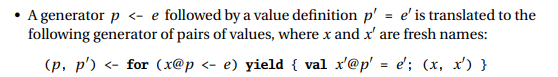# Surprising scala for loop evaluation

After working 2 years in scala I stumbled upon a surprising implementation detail of scala for loops.
To be honest, to me this looks like a bug.

Concretely the scala specification of for loops states thatwhich essentially introduces a new (unexpected) for loop.

Let me illustrate a bit more what this means. Take the following code as an example

``````for{
i <- 1 to 3
_ = println(s"i = \$i")
js = i to 3
_ = println(js)
j <- js
_ = println(s"j = \$j")
} println((i, j))
``````

What would you guess the output looks like? At least I myself was very surprised finding it to be

``````i = 1
Range(1, 2, 3)
i = 2
Range(2, 3)
i = 3
Range(3)
j = 1
j = 2
j = 3
(1,1)
(1,2)
(1,3)
j = 2
j = 3
(2,2)
(2,3)
j = 3
(3,3)
``````

The problem is that the side-effects are executed at an non-intuitive place in time. To my honest opinion, this is 100% unwanted behaviour, as it will lead to many subtle bugs.

To get the expected behaviour the collections need to be cast to Streams

``````for{
i <- (1 to 3).toStream
_ = println(s"i = \$i")
js = (i to 3).toStream
_ = println(js)
j <- js
_ = println(s"j = \$j")
} println((i, j))
``````

this will indeed output

``````i = 1
Stream(1, ?)
j = 1
(1,1)
j = 2
(1,2)
j = 3
(1,3)
i = 2
Stream(2, ?)
j = 2
(2,2)
j = 3
(2,3)
i = 3
Stream(3, ?)
j = 3
(3,3)
``````

To finish this contribution, the change needed for this is not magic at all, it just needs a different code rewrite. Concretely, all normal value definitions need to be included in the scope of the nested code block.
The value definition must be included right after `=>` respectively:

• [here should be images of how a normal for loop without value definitions is translated to `foreach`, `map` and `flatMap`, however I am to newbie to be able to upload more than one image per topic, awesome]
• hence please look them up yourself on page 89/90 of https://www.scala-lang.org/docu/files/ScalaReference.pdf

Any comments on why this was implemented this way are highly welcome
All endorsement to get such an adaptation into Scala 3 are also highly welcome

In practice the semantics of `for` depend on the implementation of `foreach`, `map`, and `flatMap`, and I suspect what you’re seeing is a result of that. If you want specific evaluation order you’d have to pick a collection with that evaluation order. For instance, I could make a collection whose `foreach` function goes in reverse order, and you wouldn’t want to use it here.
For fun, I wanted to see what `par` did. If you suffix your ranges with `par`, evaluation hangs. Surprising indeed.

were you in the REPL? see https://github.com/scala/bug/issues/8119

The desugaring of imperative for comprehensions is downright awful. I suspect it’s done this for consistency with `yield`ing comprehensions, but I think it’s a bad idea and has terrible performance implications (as well as surprises for users).

I proposed to change it here, but got no answers.

4 Likes

Yes I was in the REPL. I’m glad it’s a known problem, and the thread you linked helped me get it working. I wrapped the `for` in a method to get around the static initialization deadlock.